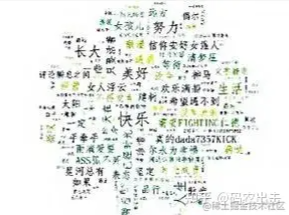﻿ Python实现微信好友数据爬取及分析_python_脚本之家
python# Python实现微信好友数据爬取及分析

## 前言• itchat：微信网页版接口封装Python版本，在本文中用以获取微信好友信息。
• jieba：结巴分词的 Python 版本，在本文中用以对文本信息进行分词处理。
• matplotlib：Python 中图表绘制模块，在本文中用以绘制柱形图和饼图
• snownlp：一个 Python 中的中文分词模块，在本文中用以对文本信息进行情感判断。
• PIL：Python 中的图像处理模块，在本文中用以对图片进行处理。
• numpy：Python中 的数值计算模块，在本文中配合 wordcloud 模块使用。
• wordcloud：Python 中的词云模块，在本文中用以绘制词云图片。
• TencentYoutuyun：腾讯优图提供的 Python 版本 SDK ，在本文中用以识别人脸及提取图片标签信息。

## 1.数据分析

```itchat.auto_login(hotReload = True)
friends = itchat.get_friends(update = True)```## 2.好友性别

```def analyseSex(firends):
sexs = list(map(lambda x:x['Sex'],friends[1:]))
counts = list(map(lambda x:x,Counter(sexs).items()))
labels = ['Unknow','Male','Female']
colors = ['red','yellowgreen','lightskyblue']
plt.figure(figsize=(8,5), dpi=80)
plt.axes(aspect=1)
plt.pie(counts, #性别统计结果
labels=labels, #性别展示标签
colors=colors, #饼图区域配色
labeldistance = 1.1, #标签距离圆点距离
autopct = '%3.1f%%', #饼图区域文本格式
startangle = 90, #饼图起始角度
pctdistance = 0.6 #饼图区域文本距离圆点距离
)
plt.legend(loc='upper right',)
plt.title(u'%s的微信好友性别组成' % friends['NickName'])
plt.show()
```## 3.好友头像

```def analyseHeadImage(frineds):
# Init Path
basePath = os.path.abspath('.')
if(os.path.exists(baseFolder) == False):
os.makedirs(baseFolder)
# Analyse Images
faceApi = FaceAPI()
use_face = 0
not_use_face = 0
image_tags = ''
for index in range(1,len(friends)):
friend = friends[index]
imgFile = baseFolder + '\\Image%s.jpg' % str(index)
if(os.path.exists(imgFile) == False):
with open(imgFile,'wb') as file:
file.write(imgData)
# Detect Faces
time.sleep(1)
result = faceApi.detectFace(imgFile)
if result == True:
use_face += 1
else:
not_use_face += 1
# Extract Tags
result = faceApi.extractTags(imgFile)
image_tags += ','.join(list(map(lambda x:x['tag_name'],result)))
labels = [u'使用人脸头像',u'不使用人脸头像']
counts = [use_face,not_use_face]
colors = ['red','yellowgreen','lightskyblue']
plt.figure(figsize=(8,5), dpi=80)
plt.axes(aspect=1)
plt.pie(counts, #性别统计结果
labels=labels, #性别展示标签
colors=colors, #饼图区域配色
labeldistance = 1.1, #标签距离圆点距离
autopct = '%3.1f%%', #饼图区域文本格式
startangle = 90, #饼图起始角度
pctdistance = 0.6 #饼图区域文本距离圆点距离
)
plt.legend(loc='upper right',)
plt.title(u'%s的微信好友使用人脸头像情况' % friends['NickName'])
plt.show()
image_tags = image_tags.encode('iso8859-1').decode('utf-8')
back_coloring = np.array(Image.open('face.jpg'))
wordcloud = WordCloud(
font_path='simfang.ttf',
background_color="white",
max_words=1200,
max_font_size=75,
random_state=45,
width=800,
height=480,
margin=15
)
wordcloud.generate(image_tags)
plt.imshow(wordcloud)
plt.axis("off")
plt.show()
```## 4.好友签名

```def analyseSignature(friends):
signatures = ''
emotions = []
pattern = re.compile("1f\d.+")
for friend in friends:
signature = friend['Signature']
if(signature != None):
signature = signature.strip().replace('span', '').replace('class', '').replace('emoji', '')
signature = re.sub(r'1f(\d.+)','',signature)
if(len(signature)>0):
nlp = SnowNLP(signature)
emotions.append(nlp.sentiments)
signatures += ' '.join(jieba.analyse.extract_tags(signature,5))
with open('signatures.txt','wt',encoding='utf-8') as file:
file.write(signatures)
# Sinature WordCloud
back_coloring = np.array(Image.open('flower.jpg'))
wordcloud = WordCloud(
font_path='simfang.ttf',
background_color="white",
max_words=1200,
max_font_size=75,
random_state=45,
width=960,
height=720,
margin=15
)
wordcloud.generate(signatures)
plt.imshow(wordcloud)
plt.axis("off")
plt.show()
wordcloud.to_file('signatures.jpg')
# Signature Emotional Judgment
count_good = len(list(filter(lambda x:x>0.66,emotions)))
count_normal = len(list(filter(lambda x:x>=0.33 and x<=0.66,emotions)))
labels = [u'负面消极',u'中性',u'正面积极']
plt.rcParams['font.sans-serif'] = ['simHei']
plt.rcParams['axes.unicode_minus'] = False
plt.xlabel(u'情感判断')
plt.ylabel(u'频数')
plt.xticks(range(3),labels)
plt.legend(loc='upper right',)
plt.bar(range(3), values, color = 'rgb')
plt.title(u'%s的微信好友签名信息情感分析' % friends['NickName'])
plt.show()
```## 5.好友位置

```def analyseLocation(friends):
with open('location.csv','w',encoding='utf-8',newline='',) as csvFile:
for friend in friends[1:]:
row = {}
row['NickName'] = friend['NickName']
row['Province'] = friend['Province']
row['City'] = friend['City']
writer.writerow(row)
```